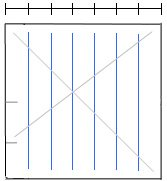# Divide Paper into Sevenths: Method 3This method of dividing a square sheet of paper into 7 equal segments leaves 4 crease lines. Diagram is from J Montroll’s book, Origami Polyhedra Design.#### Divide Paper into Sevenths: Method 3

• Fold and unfold a square sheet of paper in half making a horizontal crease. You can crease across the entire sheet or make a pinch-crease at the left edge of the sheet.
• Fold and unfold the bottom half of the paper; make a pinch-crease at the 1/4 mark.
• Fold and unfold along the diagonal from the top-right corner of the paper to the 1/4 pinch-crease.
• Fold and unfold in half along the diagonal (bring top-right corner to meet with bottom-left corner).
• The location where the two diagonal creases meet marks the 3/7 and 4/7 division. Make a fold/unfold here.
• Fold the 4/7 section in half to achieve 2/7 divisions.
• Fold the 2/7 divisions in half to achieve 1/7.
• Use this 1/7 division to fold the remaining section of the paper into equal 1/7 divisions.Note: the point where the two diagonal lines intersect marks the 3/7 division along the vertical and the horizontal. Thus, a vertical crease or a horizontal crease which passes through the dot gives 3/7 and 4/7 divisions.